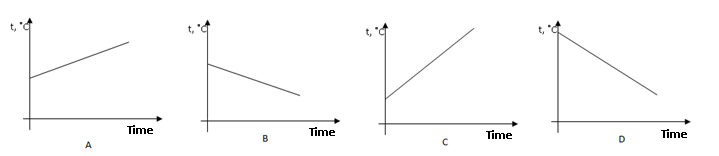##### KAPEC GEOTASK?
• The learning process is entertaining and exciting
• The solved tasks turn into coordinates
• The chance to follow your progress
• Along with your schoolmates and friends, go on an adventure, searching for hideouts
• E-mail:

# 112.2. Heat process diagram.

#### Check the correct answer!In equal bowls are different liquids, but their masses are equal. All bowl can be cooled and heated in equal conditions. Any liquid (A, B, C, and D) temperature changes are illustrated in different graphics. All function times and temperature scales are equal. Which liquid is cooled?

#### Next task: 1345. Heat process diagram.

Grade:
8
Subject
Physics
Theme
Heat process
Subtheme
Heat process diagram.

#### Solve tasks to find hidden Geo point coordinates

1. Algebra: Quadratic equation
2. Algebra: Quadratic equation discriminant
3. Chemistry: Air composition and usage(reactions).
4. Geometry: Tangent properties and signs.
5. Algebra: Quadratic equation discriminant
6. Chemistry: Substance oxidation process and reactions.
7. Algebra: ax² + c = 0
8. Algebra: Viet theorem.
9. Geometry: Squar, properties and signs.
10. Algebra: Median